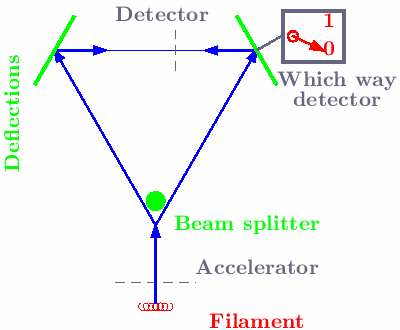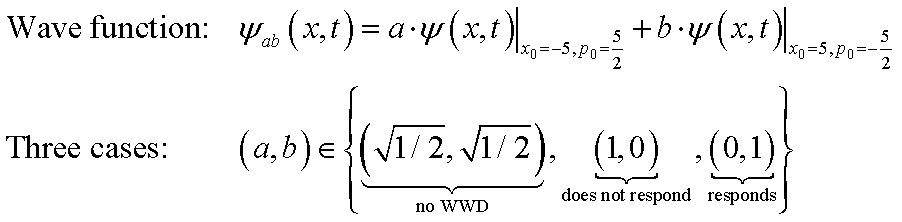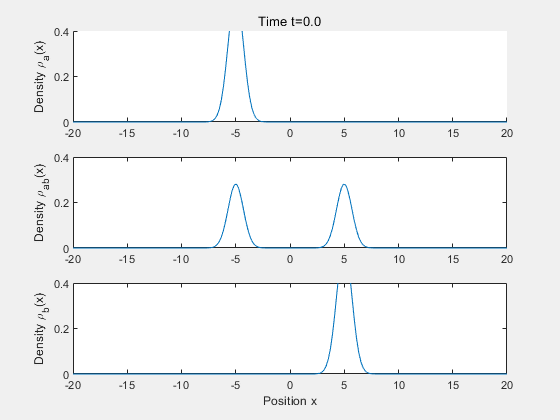Institut für Theoretische Physik

## Basic Physics Course with MATLAB's Symbolic Toolbox and Live Editor

### Animation 5: Gauss interference

 A beam with action quanta is split and superimposed by deflections again. For simplification, the probability density of detection is calculated only after the deflections. Three cases are distinguished in the wave function (see below): • No which-way detector (WWD) • WWD does not respond • WWD responds The wave function ψ(x,t) of a Gaussian wave packet is parametrized by x0 and p0, so that it has the mean values at time t=0 for space and momentum: ˂x˃=x0 and ˂p˃=p0.The upper figure showns the time evolution of the probability density of detection ρ=|ψ|2 if the WWD does not respond.
The figure below showns the time evolution of the probability density of detection ρ=|ψ|2 if the WWD responds.
The middle figure showns the time evolution of the probability density of detection ρ=|ψ|2 if there is no WWD. In this case quantum interference is observed. This shows very clearly the difference between particles and action quanta.
Note that in "particle physics" action quanta and not particles are considered!
Further details can be found in Chap05_3 .